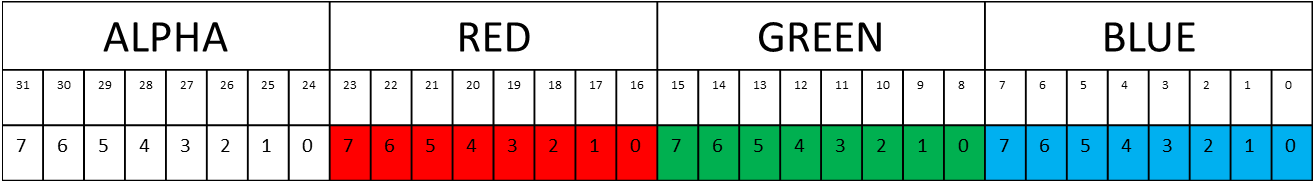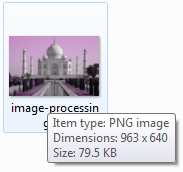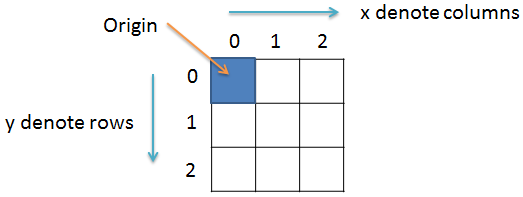# How to get and set pixel value in Java

Image Processing Project

In this theory part of the Image Processing Project we will learn about pixels. We will learn how to store pixel value in a variable. How to get pixel values of an image and how to set pixel value of an image in Java programming language.

### Pixel

The smallest unit of an image is called a Pixel, and it is generally made up of 4 components namely:

• Alpha
• Red
• Green
• Blue

Alpha determines the transparency while Red, Green and Blue determines the color of the pixel. We generally denote these four components as A for Alpha, R for Red, G for Green and B for Blue.

Each of these four components (ARGB) take a value between 0 to 255 both inclusive, where 0 means the component is missing and 255 means the component is fully present.

Since, 28 = 256. So, 8 bits can represent any value in the range 0 to 255. This means we will need only 8 bits to store the value of any of the four component. And since a pixel has 4 components so, the total number of bits required to store the value of all the four components ARGB is equal to 4x8 = 32 bits or 4 bytes.

The image shown below represent a single pixel value consisting of 32 bits. Alpha take the leftmost 8 bits while Blue takes the rightmost 8 bits of the pixel. The first bit is at the rightmost side and is numbered or indexed 0 and the last bit is at the leftmost side and is numbered or indexed 31.The bit position of the 4 components is summarized in the table below

 ALPHA RED GREEN BLUE BIT POSITION 31 - 24 23 - 16 15 - 8 7 - 0

Each of these bit position will take any of the two bit values or binary values i.e., 0 or 1.

Therefore, if all the 8 bits of ALPHA is set to 0 i.e., 0000 0000. Then converting this value into decimal, we will get (0000 0000)2 = 010

Similarly, if all the 8 bits of ALPHA is set to 1 i.e., 1111 1111. Then converting this value into decimal, we will get (1111 1111)2 = 25510

We can also represent the bit values in hexadeimal form. So, (0000 0000)2 in binary is equal to (00)16in hexadecimal. Similarly, (1111 1111)2 in binary is equal to (FF)16 in hexadecimal.

### 2D Images

2D images have a width and a height generally denoted in pixels. In the following image we get the detail like Type, Dimensions and Size of the Image File.Dimensions of an image file is generally represented in width x height format. In the above picture we can see the dimensions as 963x640. That is the width of the image is 963 pixels and the height of the image is 640 pixels.

### Representing 2D image pixels

For a 2D image we will have pixels arranged in rows and columns. Origin (starting pixel) of the image is at the coordinate (0,0).

The image show below has dimensions 3x3.So, we can denote a pixel (x,y) as Px,y(A,R,G,B)

Where, (x,y) is the co-ordinate of the pixel and A, R, G and B denote the Alpha, Red, Green and Blue value of the pixel respectively.

Example: P0,0(255,100,150,200) represents a pixel at co-ordinate (0,0) having A = 255, R = 100, G = 150 and B = 200.

### Prerequisite

To proceed further it is assumed that you have completed the previous project How to read and write image file in Java

### Code we will use in this project

``import java.io.File;``import java.io.IOException;``import java.awt.image.BufferedImage;``import javax.imageio.ImageIO;``
``public class GetSetPixels{``  public static void main(String args[])throws IOException{``    BufferedImage img = null;``    File f = null;``    //read image``    try{``      f = new File("D:\\Image\\Sample.jpg");``      img = ImageIO.read(f);``    }catch(IOException e){``      System.out.println(e);``    }    // some code goes here...``  }//main() ends here``}//class ends here``

In the above code we have imported the required classes. Then inside the main() function we are reading the Sample.jpg image file.

The File object f holds the image file path "D:\\Image\\Sample.jpg". The Sample.jpg image is of dimensions 1x1, i.e., it is a single pixel image.

``f = new File("D:\\Image\\Sample.jpg");``

Then we are reading the image and storing it in variable img.

``img = ImageIO.read(f);``

### How to get image dimensions?

To get the image dimensions we use the getWidth() and getHeight() functions. We will create two integer variables width and height for this purpose.

``int width = img.getWidth();int height = img.getHeight();``

Since, the Sample.jpg is a single pixel image so, variable width and height each will hold value 1.

Note! We will not be using the width and height variable value in this project. But this concept will be used in the later projects.

### How to get the pixel value?

To get the pixel value we use the getRGB(x,y) function. This function takes as parameter the co-ordinate of the pixel and it returns an integer value which can be positive and negative. So, to store the pixel value we will create an integer variable.

``int p = img.getRGB(x,y);``

As, Sample.jpg is a single pixel image so, to read that pixel we will replace (x,y) with (0,0) which is the co-ordinate of that pixel.

So, the above code will be written as:

``int p = img.getRGB(0,0);``

### How to get ALPHA, RED, GREEN and BLUE value of the pixel?

So, we have the pixel value stored in the integer variable p it's time for us to get each of the four component (ARGB) values. And since we know that ARGB value is an integer in the range 0 to 255 so, we will create 4 integer variables a, r, g and b for this purpose.

We, have seen that the ALPHA bits occupy 8 bits from index 24 to index 31. So, to get the ALPHA bits we have to first right shift the 32 bits of the pixels by 24 position and then bitwise ADD it with 0xFF.

When we perform the right shift operation we bring the bits to the rightmost 8 bit position. And when we bitwise ADD it with 0xFF we are left with the value of the component we are interested in.

So, to get the required Alpha value we will write:

``int a = (p>>24) & 0xff;``

Note! 0xFF is the hexadecimal representation of the decimal value 255.

Similarly, the RED bits occupy 8 bits from index 16 to index 23. So to get the RED bits we have to first right shift the 32 bits of the pixels by 16 position and then bitwise ADD it with 0xFF. For this we will write:

``int r = (p>>16) & 0xff;``

Likewise, the GREEN bits occupy 8 bits from index 8 to index 15. So, to get the GREEN bits we have to right shift the 32 bits of the pixels by 8 positionand then bitwise ADD it with 0xFF.

``int g = (p>>8) & 0xff;``

And, to get the BLUE bits which occupy 8 bits from index 0 to index 7 we DON'T have to right shift the pixel bits. This is because the BLUE bits already occupy the rightmost 8 bits. So, we will simply bitwise ADD the pixel value with 0xFF.

``int b = p & 0xff;``

Now, our code will look something like the following.

``import java.io.File;``import java.io.IOException;``import java.awt.image.BufferedImage;``import javax.imageio.ImageIO;``
``public class GetSetPixels{``  public static void main(String args[])throws IOException{``    BufferedImage img = null;``    File f = null;``    //read image``    try{``      f = new File("D:\\Image\\Sample.jpg");``      img = ImageIO.read(f);``    }catch(IOException e){``      System.out.println(e);``    }    //get image width and height    int width = img.getWidth();    int height = img.getHeight();    /**     * Since, Sample.jpg is a single pixel image so, we will     * not be using the width and height variable in this     * project.     */    //get pixel value    int p = img.getRGB(0,0);    //get alpha    int a = (p>>24) & 0xff;    //get red    int r = (p>>16) & 0xff;    //get green    int g = (p>>8) & 0xff;    //get blue    int a = p & 0xff;    //some code goes here...``  }//main() ends here``}//class ends here``

### How to set the pixel value?

To keep the project simple, lets set the value of Alpha, Red, Green and Blue to 255, 100, 150 and 200 respectively. For this we will write.

``a = 255;r = 100;g = 150;b = 200;``

To set the pixel value with the new ARGB value we will first set p to 0.

``p = 0;``

Note! p is an integer variable and holds the value of the pixel.

We, know that 8 bits of the ALPHA component occupy the bit position from index 24 to index 31. So, we will left shift the alpha value by 24 position and bitwise OR it with the p value.

``p = p | (a<<24);``

Similarly, 8 bits of the RED component occupy the bit position from index 16 to index 23. So, we will left shift the red value by 16 position and bitwise OR it with the p value.

``p = p | (r<<16);``

Likewise, 8 bits of the GREEN component occupy the bit position from index 8 to index 15. So, we will left shift the green value by 8 position and bitwise OR it with the p value.

``p = p | (g<<8);``

And for BLUE component we DON'T have to left shift. We, will simply bitwise OR it with p.

``p = p | b;``

We, can write the above lines of code in a single line as:

``p = (a<<24) | (r<<16) | (g<<8) | b;``

To set this pixel value to the image at co-ordinate (x,y) we use the setRGB(x,y,p) function. And Sample.jpg image is a single pixel image so, we will replace (x,y) with (0,0).

For this we will write:

``img.setRGB(0, 0, p);``

### Final code

``import java.io.File;``import java.io.IOException;``import java.awt.image.BufferedImage;``import javax.imageio.ImageIO;``
``public class GetSetPixels{``  public static void main(String args[])throws IOException{``    BufferedImage img = null;``    File f = null;``    //read image``    try{``      f = new File("D:\\Image\\Sample.jpg");``      img = ImageIO.read(f);``    }catch(IOException e){``      System.out.println(e);``    }    //get image width and height    int width = img.getWidth();    int height = img.getHeight();    /**     * Since, Sample.jpg is a single pixel image so, we will     * not be using the width and height variable in this     * project.     */    //get pixel value    int p = img.getRGB(0,0);    //get alpha    int a = (p>>24) & 0xff;    //get red    int r = (p>>16) & 0xff;    //get green    int g = (p>>8) & 0xff;    //get blue    int a = p & 0xff;    /**     * to keep the project simple we will set the ARGB     * value to 255, 100, 150 and 200 respectively.     */    a = 255;    r = 100;    g = 150;    b = 200;    //set the pixel value    p = (a<<24) | (r<<16) | (g<<8) | b;    img.setRGB(0, 0, p);    //write image    try{      f = new File("D:\\Image\\Output.jpg");      ImageIO.write(img, "jpg", f);    }catch(IOException e){      System.out.println(e);    }``  }//main() ends here``}//class ends here``